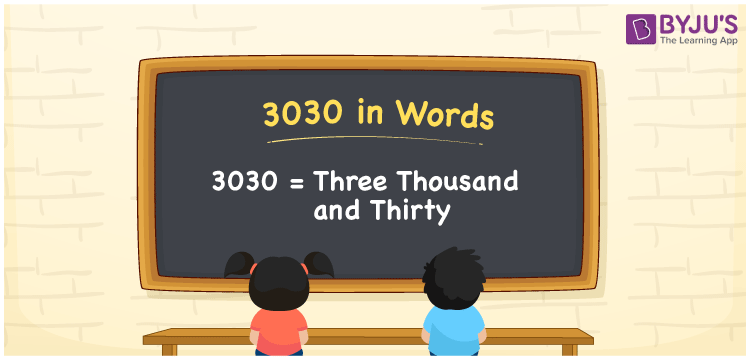# 3030 in Words

The number 3030 in words is represented by Three Thousand and Thirty. We use the ones, tens, hundreds, thousands places to write the number name of any number. Thus, a place value chart is very useful in converting the number into the word form. For example, if you purchased a table fan worth Rs. 3030, then you can say, “I purchased a table fan worth Rupees Three Thousand and Thirty”. 3030 is a cardinal number since it shows a specific amount.

 3030 in Words Three Thousand and Thirty Three Thousand and Thirty in numerical form 3030

## 3030 in English Words

In Maths, students write the numbers in words using the English alphabet. Therefore, the number 3030 in English is written as Three Thousand and Thirty.## How to Write 3030 in Words?

Find the position of each digit in the number 3030 before writing it in word form. The following is the place value chart for the number 3030.

 Thousands Hundreds Tens Ones 3 0 3 0

Hence, we can write the expanded form as:

3 x Thousand + 0 x Hundred + 3 x Ten + 0 x One

= 3 x 1000 + 0 x 100 + 3 x 10 + 0 x 1

= 3000 + 0 + 30 + 0

= 3000 + 30

= 3030

= Three Thousand and Thirty

Therefore, 3030 in words is written as Three Thousand and Thirty

Interesting way of writing 3030 in words

3 = Three

30 = Thirty

303 = Three Hundred and Three

3030 = Three Thousand and Thirty

Thus, the word form of the number 3030 is Three Thousand and Thirty

3030 is a natural number that is the successor of 3029 and the predecessor of 3031

• 3030 in words – Three Thousand and Thirty
• Is 3030 an odd number? – No
• Is 3030 an even number? – Yes
• Is 3030 a perfect square number? – No
• Is 3030 a perfect cube number? – No
• Is 3030 a prime number? – No
• Is 3030 a composite number? – Yes

## Frequently Asked Questions on 3030 in Words

Q1

### Write 3030 in words.

3030 in words is written as Three Thousand and Thirty.
Q2

### What is the value of 4000 – 970?

4000 – 970 = 3030 Therefore, the value of 4000 – 970 is 3030.
Q3

### Is 3030 a composite number?

Yes, 3030 is a composite number.